## Logical Conditions in Optimization

Conditional statements can cause problems for gradient-based optimization algorithms because they are often posed in a form that gives discontinuous functions, first derivatives, or second derivatives.

For example, the absolute value operator is a continuous function but gives a discontinuous first derivative at the origin.Minimizing this function can cause a problem for solvers that rely on first and second derivative information to determine search directions at each iteration. As an exercise, solve the following model that uses the absolute value operator.

from gekko import GEKKO

m = GEKKO()
x = m.Param(-1)
# use abs to define a new variable
y = m.abs(x)
# use abs in an equation
z = m.Var()
m.Equation(z==m.abs(x)+1)

# solve
m.solve()

print('x: ' + str(x.value))
print('y: ' + str(y.value))
print('z: ' + str(z.value))

The solver can still converge because the solution is away from the discontinuity. In Example 2, the value of x is now determined by the optimizer. The objective function to minimize (y+3)2 means that the optimal solution is at x=0 and y=0. However, when the solution is at the discontinuity, a gradient based solver will often fail to converge.

Python GEKKO ABS function in optimization (Failure)

from gekko import GEKKO
# define new GEKKO model
m = GEKKO()
# calculate x to minimize objective
x = m.Var(1)
# use abs to define y
y = m.abs(x)
# define objective to minimize
m.Minimize((y+3)**2)
# solve
m.solve()
# print solution
print('x: ' + str(x.value))
print('y: ' + str(y.value))

In this case, the IPOPT solver reaches the default maximum number of iterations (100) and reports a failure to converge. However, if another form with continuous first and second derivatives (abs2 in GEKKO) is used, a solution is found.

Python GEKKO ABS2 function in optimization (Success)

from gekko import GEKKO
# define new GEKKO model
m = GEKKO()
# calculate x to minimize objective
x = m.Var(1)
# use abs2 to define y
y = m.abs2(x)
# define objective to minimize
m.Minimize((y+3)**2)
# solve
m.solve()
# print solution
print('x: ' + str(x.value))
print('y: ' + str(y.value))

Also, if the problem is reformulated with an additional binary variable intb, the optimizer can find a solution to the same problem that failed with the abs(x) operator. The value of intb is a binary variable that can be either 0 or 1. The value of intb is zero when x<0 and is one when x>=0. The following two examples show that this reformulation allows the solver to quickly find a solution either away from or at the discontinuity.

Python GEKKO binary variable in simulation (Success)

from gekko import GEKKO
# define new GEKKO model
m = GEKKO()
# parameter
x = m.Param(-0.5)
# define new binary variable
intb = m.Var(0,lb=0,ub=1,integer=True)
# define y
y = m.Var()
# define equations
m.Equation((1-intb)*x <= 0)
m.Equation(intb * (-x) <= 0)
# output
m.Equation(y==(1-intb)*(-x) + intb*x)
# solve with APOPT (MINLP solver)
m.options.SOLVER=1
m.solve()
# print solution
print('x: ' + str(x.value))
print('intb: ' + str(intb.value))
print('y: ' + str(y.value))
# with abs3 object
from gekko import GEKKO
# define new GEKKO model
m = GEKKO()
# parameter
x = m.Param(-0.5)
# calculate y=abs(x) with abs3
y = m.abs3(x)
# solve with APOPT (MINLP solver)
m.solve()
# print solution
print('x: ' + str(x.value))
print('y: ' + str(y.value))

Python GEKKO binary variable in optimization (Success)

from gekko import GEKKO
# define new GEKKO model
m = GEKKO()
# calculate x to minimize objective
x = m.Var(1.0)
# define new binary variable
intb = m.Var(0,lb=0,ub=1,integer=True)
# define y
y = m.Var()
# define equations
m.Equation((1-intb)*x <= 0)
m.Equation(intb * (-x) <= 0)
# output
m.Equation(y==(1-intb)*(-x) + intb*x)
# solve with APOPT (MINLP solver)
m.options.SOLVER=1
m.solve()
# print solution
print('x: ' + str(x.value))
print('intb: ' + str(intb.value))
print('y: ' + str(y.value))
# with abs3 object
from gekko import GEKKO
# define new GEKKO model
m = GEKKO()
# variable
x = m.Var(-0.5)
# calculate y=abs(x) with abs3
y = m.abs3(x)
# solve with APOPT (MINLP solver)
m.solve()
# print solution
print('x: ' + str(x.value))
print('y: ' + str(y.value))

The above examples demonstrate this concept with the absolute value operator. The same techniques can be applied to:

• If...Else Statements
• Max Function (see Python GEKKO max2 and max3 functions)
• Min Function (see Python GEKKO min2 and min3 functions)
• Minimax and Maximin
• Piece-wise Linear Functions (see Python GEKKO pwl function)
• Signum Function (What is Signum?)
• Other discontinuous functions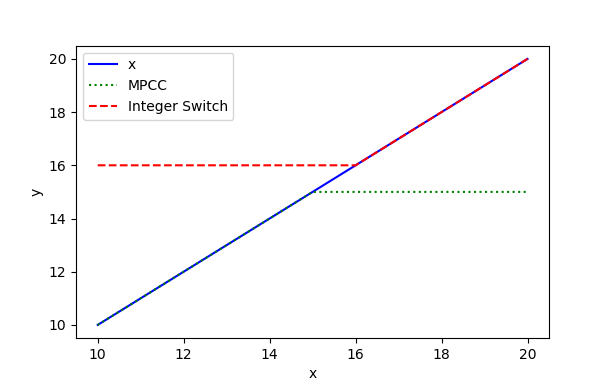import numpy as np
import matplotlib.pyplot as plt
from gekko import GEKKO
m = GEKKO(remote=False)
p = m.Param(value=np.linspace(10,20,21))
x = m.Var()
m.Equation(x==p)
# with MPCCs
y2 = m.min2(p,15)
# with integer variables
y3 = m.max3(p,16)
m.options.IMODE = 2
m.solve()
plt.plot(p,x,'b-',label='x')
plt.plot(p,y2,'g:',label='MPCC')
plt.plot(p,y3,'r--',label='Integer Switch')
plt.legend()
plt.xlabel('x')
plt.ylabel('y')
plt.show()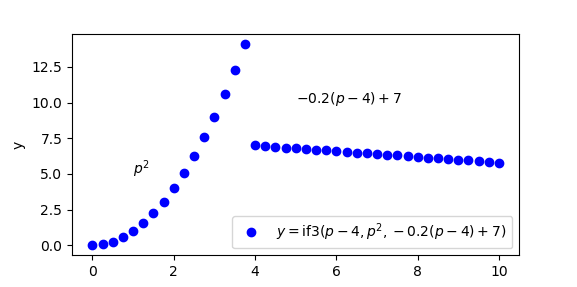import numpy as np
import matplotlib.pyplot as plt
from gekko import GEKKO
m = GEKKO(remote=False)
p = m.Param(value=np.linspace(0,10,41))
# conditional statement
y = m.if3(p-4,p**2,-0.2*(p-4)+7)
m.options.IMODE = 2
m.solve()
lbl = r'$y=\mathrm{if3}(p-4,p^2,-0.2(p-4)+7)$'
plt.plot(p,y,'bo',label=lbl)
plt.text(1,5,r'$p^2$')
plt.text(5,10,r'$-0.2 (p-4)+7$')
plt.legend(loc=4)
plt.ylabel('y')
plt.show()

Two popular methods for reformulation of the conditional statements is to either use:

• Binary or integer variables
• Mathematical Programs with Equilibrium/Complementarity Constraints (MPECs/MPCCs)

Each of these techniques are described below with a number of examples.

#### Logical Conditions with Binary Variables

The approach taken above with the ABS function used a single binary variable.

The following examples demonstrate the use of conditional statements to switch between different functions. In this case, the function y=6*exp(-x)+1 if the value of x<3 else the function y=x is chosen when x>=3. The simulate example verifies that the correct value is reported with a fixed value of x. The second example shows how x can be adjusted to find a minimum value of y or f(x).

from gekko import GEKKO
m = GEKKO()
x = m.Param(0.5)
y = m.Var()
m.Equation(y==m.if3(x-3,6*m.exp(-x)+1,x))
m.solve()
print(x.value,y.value)

from gekko import GEKKO
m = GEKKO()
x = m.Var(0.5)
y = m.if3(x-3,6*m.exp(-x)+1,x)
m.Minimize(y)
m.solve()
print(x.value,y.value)With three functions an if...elseif...else structure may be used. The following two examples show how to implement these conditional statements to achieve continuous first and second derivatives.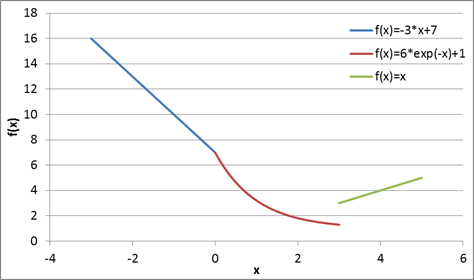Piece-wise Linear (PWL) functions are one method to approximate any nonlinear function. The following example programs demonstrate a piece-wise linear function with binary decision variables. The sum of the binary decision variables is required to be one, meaning that only one of the linear approximations can be active at a time.

The value of x is now declared as a decision variable and is adjusted by the optimizer to minimize the objective function that is equal to y. By inspection, the optimal value is at x=2 giving a result of y=0.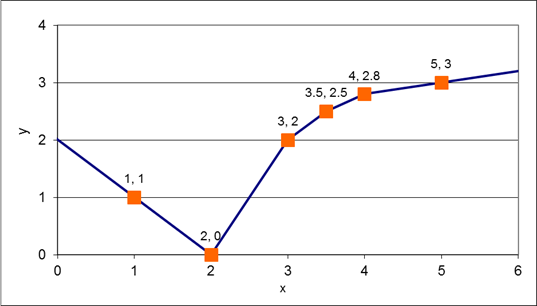The example shown above demonstrates a PWL function with one input and one output but it can also be extended to cases with multiple inputs and multiple outputs.

#### MPCCs: Mathematical Programs with Complementarity Constraints

Mathematical Programs with Complementarity Constraints (MPCCs) are formulations that can also be used to model certain classes of discrete events. MPCCs can be more efficient than solving mixed integer formulations of the optimization problems because it avoids the combinatorial difficulties of searching for optimal discrete variables.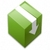Sine Wave Clipping